1596737040

# Algorithms From Scratch: Support Vector Machine

A popular algorithm that is capable of performing linear or non-linear classification and regression, Support Vector Machines were the talk of the town before the rise of deep learning due to the exciting kernel trick — If the terminology makes no sense to you right now don’t worry about it. By the end of this post you’ll have an good understanding about the intuition of SVMs, what is happening under the hood of linear SVMs, and how to implement one in Python.

To see the full Algorithms from Scratch Series click on the link below.

## Algorithms From Scratch - Towards Data Science

Read writing about Algorithms From Scratch in Towards Data Science. A Medium publication sharing concepts, ideas, and…

towardsdatascience.com

## Intuition

In classification problems the objective of the SVM is to fit the largest possible margin between the 2 classes. On the contrary, regression task flips the objective of classification task and attempts to fit as many instances as possible within the margin — We will first focus on classification.

If we focus solely on the extremes of the data (the observations that are on the edges of the cluster) and we define a threshold to be the mid-point between the two extremes, we are left with a margin that we use to sepereate the two classes — this is often referred to as a hyperplane. When we apply a threshold that gives us the largest margin (meaning that we are strict to ensure that no instances land within the margin) to make classifications this is called Hard Margin Classification (some text refer to this as Maximal Margin Classification).

When detailing hard margin classification it always helps to see what is happening visually, hence Figure 2 is an example of a hard margin classification. To do this we will use the iris dataset from scikit-learn and utility function `plot_svm()` which you can find when you access the full code on github — link below.

## kurtispykes/ml-from-scratch

Permalink Dismiss GitHub is home to over 50 million developers working together to host and review code, manage…

github.com

Note: This story was written straight from jupyter notebooks using python package `_jupyter_to_medium_` — for more information on this package click here— and the committed version on github is a first draft hence you may notice some alterations to this post.

``````import pandas as pd
import numpy as np
from sklearn.svm import LinearSVC
from sklearn.preprocessing import StandardScaler
import matplotlib.pyplot as plt
%matplotlib inline
# store the data

# convert to DataFrame
df = pd.DataFrame(data=iris.data,
columns= iris.feature_names)

# store mapping of targets and target names
target_dict = dict(zip(set(iris.target), iris.target_names))

# add the target labels and the feature names
df["target"] = iris.target
df["target_names"] = df.target.map(target_dict)

# view the data
df.tail()
``````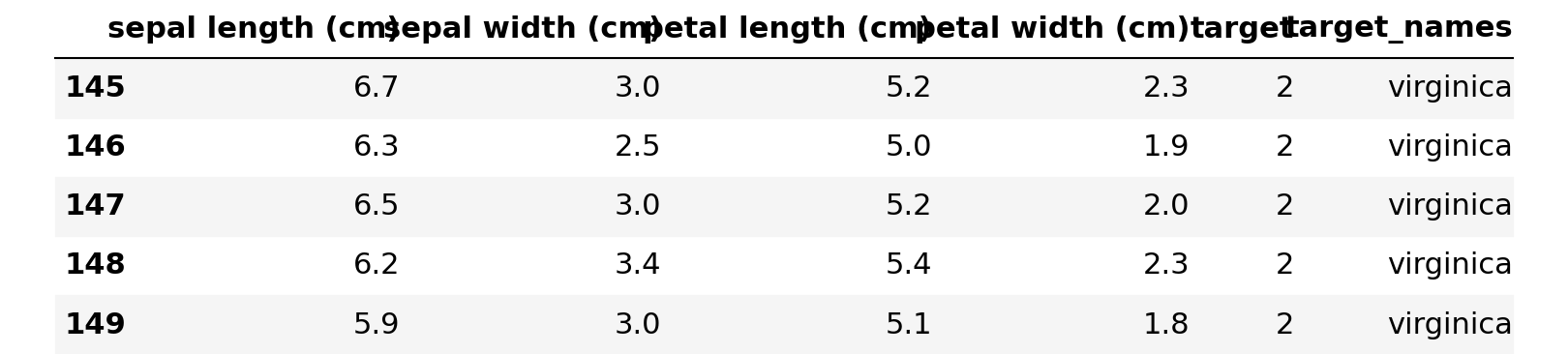Figure 1: Original Dataset

``````# setting X and y
X = df.query("target_names == 'setosa' or target_names == 'versicolor'").loc[:, "petal length (cm)":"petal width (cm)"]
y = df.query("target_names == 'setosa' or target_names == 'versicolor'").loc[:, "target"]

# fit the model with hard margin (Large C parameter)
svc = LinearSVC(loss="hinge", C=1000)
svc.fit(X, y)

plot_svm()
``````

#programming #machine-learning #data-science #artificial-intelligence #algorithms-from-scratch #algorithms

## Buddha Community1593467580

## Support Vector Machines — Thinking like vectors!

Support vector machines work well in high dimensional space with clear margin or separation thus thinking like vectors.

Support Vector Machine(SVM) is a supervised non-linear machine learning algorithm which can be used for both classification and regression problems. SVM is used to generate multiple separating hyperplanes such that it divides segments of data space and each segment contains only one kind of data.

SVM technique is useful for data whose distribution is unknown i.e which has Non-regularity i.e data in spam classification, handwriting recognition, text categorization, speaker identification etc. I listed applications of support vector machine with it.:)

This post is about explaining support vector machines with an example, demonstration of support vector machine on a dataset and explanation of generated outputs of demonstration.

# What lies behind SVM with example?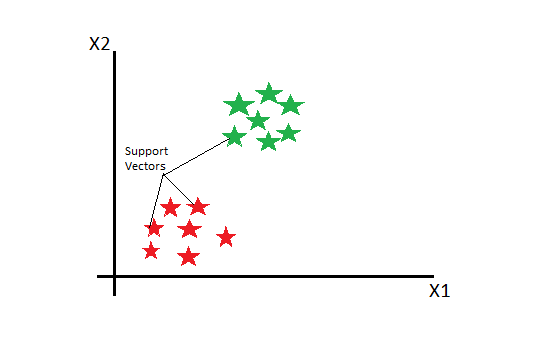Picture exclusively created

In Support Vector Machines, we plot each data as a point in n-dimensional space(where “n” is the number of features) with the value of each feature being a value of a particular coordinate. Then, we perform classification by finding hyperplane that differentiates the classes.

Example

Consider a dataset containing Apples and Oranges. So, to classify them, we use Support Vector machine ad labelled training data on plane.<

A support vector machine(SVM) takes these data points and outputs the hyperplane (which is a two-dimension line of equation y = ax + b) that best separates the tags. The line is called the **decision boundary **i.e anything that falls to one side of it is classified as Apple and anything that falls to the other as Orange.

The hyperplane(Two-dimensional line) is best when it’s the distance to the nearest element of each data point or tag is the largest i.e specified on maximum margins.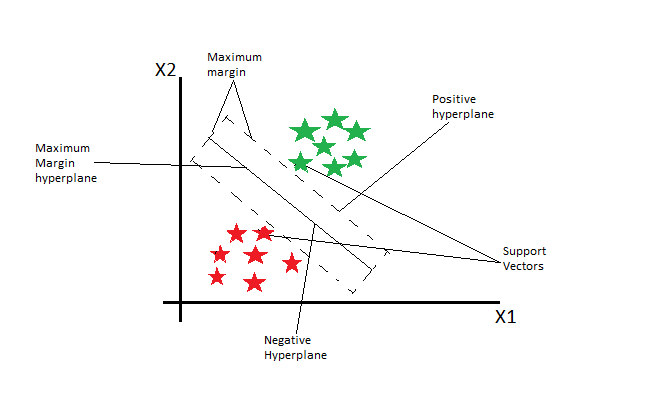All points on the line ax+b=0 will satisfy the equation so, we draw two parallel lines ax+b=-1 for one side and ax+b=1 for the other side such that these lines pass through a datapoint or tag in the segment which is nearest to our line, then the distance between these two lines will be our margin.

#algorithms #data-science #r #support-vector-machine #machine-learning #algorithms1593396341

## Support Vector Machines(SVM)-What are they?

SVM’s were initially developed in 1960s then they were refined in 1990s and now they are becoming very popular in machine learning as they are demonstrating that they are very powerful and different from other Machine Learning algorithms.

A Support Vector Machine (SVM) is a supervised machine learning algorithm that can be employed for both classification and regression purposes. They are more commonly used in classification problems.

How SVM’s Work?

Consider some usual points on a 2 dimensional space with two columns x1 & x2.

Now how can we derive a line that will separate these two different points and classify them separately? This separation or decision boundary is compulsory as when we add new points in future that we want to classify haven’t been classified yet. We will get to know whether they will fall either in Green area or Red area.

So how to separate these points?

Well there can be numerous ways of drawing lines in between that will achieve the same result as shown.

But we want to find the most optimal line that’s what SVM’s are all about. SVM’ are about finding the best decision boundary that will help us to separate out space into classes.

So lets find out how the SVM’s searches for it. The required line is searched through Maximum Margin.

We can see a line that separates these two classes of points and it has the Maximum Margin which means that the distance between the line and each of these points (touching Red and Green point) is equidistant.

Now sum of these two distances has to be maximized in order for this line to be SVM. The boundary points are know as Support Vectors. Why So?

Basically these two vectors are supporting whole algorithm rest other points don’t contribute to the result of algorithm, only these two points are contributing, therefore they are called Supporting Vectors.

#machine-learning #algorithms #svm #support-vector-machine #artificial-intelligence #algorithms1593446400

## Decoding Support Vector Machines

SVM is a very simple yet powerful supervised machine learning algorithm that can be used for classification as well as regression though its popularly used for classification. They perform really well in small to medium sized datasets and are extremely easy to tune.

In this blog post we will build our intuition of support vector machines and see some math behind it. We will first understand what large margin classifiers are and understand the loss function and the cost function of this algorithm. We will then see how regularization works for SVM and what governs the bias/variance trade off. Finally we will learn about the coolest feature of SVM, that is the Kernel trick.

You must have some pre-requisite knowledge of how linear regression and logistic regression work in order to easily grasp the concepts. I would suggest you to take notes while reading in order to make the most out of this article, it is going to be a long and interesting journey. So, without further ado lets dive in.

# Large Margin Classifier

Lets right away begin with an example, say we have some data which contains 2 classes and for simplicity lets assume it has only 2 features, we can separate these 2 classes in many different ways. We can use linear as well as non-linear decision boundaries to do so.

What SVM does is that it tries to separate these 2 classes as widely as possible and hence in our example it will choose the yellow line as its decision boundary.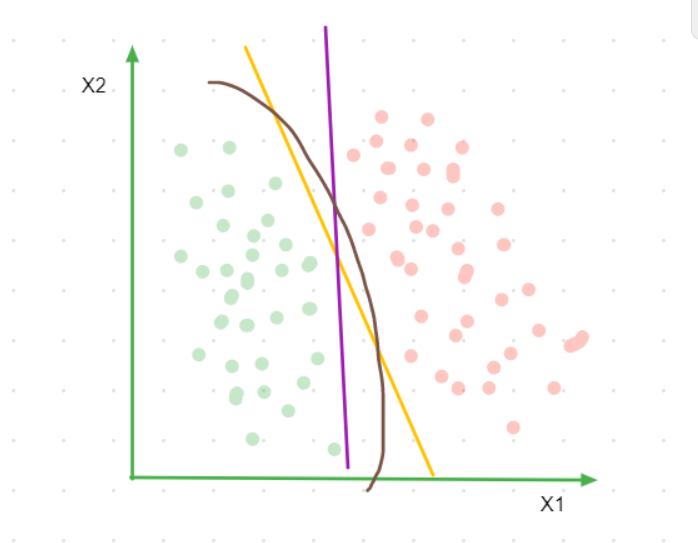Figure 1

If the yellow line is our decision boundary then the green and red class points circled (figure 2) are the closest points to our decision boundary. The distance between these points is called margin and SVM tries to maximize this margin. This is the reason why support vector machines are also called **large margin classifiers, **this enables SVM to have a better generalization accuracy.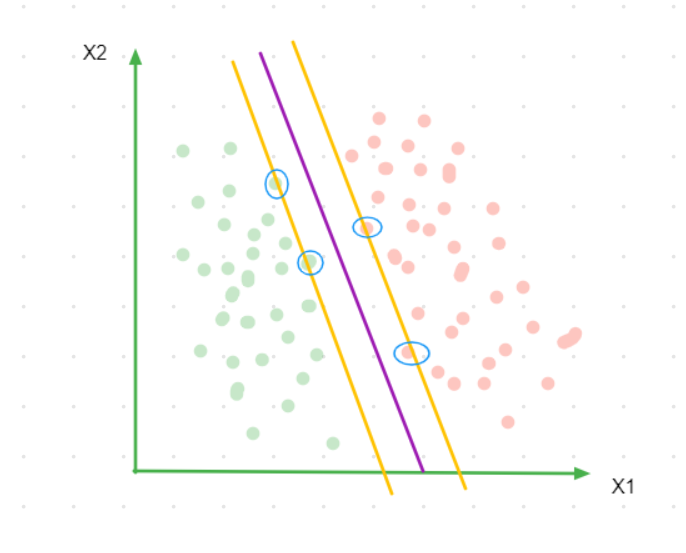Figure 2

In high dimensional space these points are nothing but n-dimensional vectors where n is the number of features in the data. A sample of points that are closest to the decision boundary (here the circled red and green points) are called support vectors. I will be calling the green support vectors as positive support vectors and the red as negative support vectors. The decision boundary is entirely dependent on these points as they are the ones which decide the length of our margin. If we change the support vectors, our decision boundary will change and that also means that points other than the support vectors don`t really matter in forming our decision boundary.

# Optimization Objective

To find the decision boundary we must :

• define our hypothesis
• define a loss function
• using the loss function calculate the cost function for all the training points
• use an optimization algorithm like gradient descent or sequential minimal optimization to minimize the cost and arrive at our ideal parameters

The hypothesis for SVM is fairly straight forward, for weights w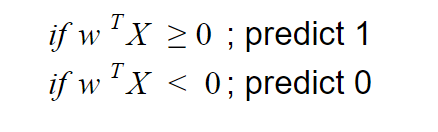Figure 3

Here a key point that you need to understand is that this hypothesis is nothing but the distance between a data point and the decision boundary, so whenever I say the word hypothesis just think of it as nothing but this distance .

Before we see what exactly the loss function is for SVM let us look at the cost for a single training example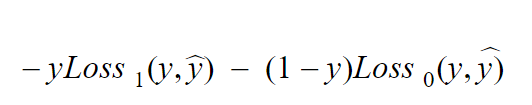Figure 4

The first term is the loss for when y = 1 and the second term is the loss when y = 0 and “y hat” is nothing but our hypothesis defined in Figure 3. I know I have given out a lot of equations don`t worry, let`s start making sense out of them.

#algorithms #classification #machine-learning #data-science #support-vector-machine #algorithms1621378980

## Support Vector Machine Algorithm in Machine Learning

Everything You Need to Know about Support Vector Machine Algorithms

Most beginners, when it comes to machine learning, start with regression and classification algorithms naturally. These algorithms are simple and easy to follow. However, it is essential to go beyond these two machine learning algorithms to grasp the concepts of machine learning better.

There is much more to learn in machine learning, which might not be as simple as regression and classification, but can help us solve various complex problems. Let us introduce you to one such algorithm, the Support Vector Machine Algorithm. Support Vector Machine algorithm, or SVM algorithm, is usually referred to as one such machine learning algorithm that can deliver efficiency and accuracy for both regression and classification problems.

If you dream of pursuing a career in the machine learning field, then the Support Vector Machine should be a part of your learning arsenal. At upGrad, we believe in equipping our students with the best machine learning algorithms to get started with their careers. Here’s what we think can help you begin with the SVM algorithm in machine learning.

### What is a Support Vector Machine Algorithm?

SVM is a type of supervised learning algorithm that has become very popular in 2020 and will continue to be so in the future. The history of SVM dates back to 1990; it is drawn from Vapnik’s statistical learning theory. SVM can be used for both regression and classification challenges; however, it is mostly used for addressing classification challenges.

SVM is a discriminative classifier that creates hyperplanes in N-dimensional space, where n is the number of features in a dataset to help discriminate future data inputs. Sounds confusing right, don’t worry, we’ll understand it in simple layman terms.

### How Does a Support Vector Machine Algorithm Work?

Before delving deep into the working of an SVM, let’s understand some of the key terminologies.

#### Hyperplane

Hyperplanes, which are also sometimes referred to as decision boundaries or decision planes, are the boundaries that help classify data points. The hyperplane’s side, where a new data point falls, can be segregated or attributed to different classes. The dimension of the hyperplane depends on the number of features that are attributed to a dataset. If the dataset has 2 features, then the hyperplane can be a simple line. When a dataset has 3 features, then the hyperplane is a 2-dimensional plane.

#### Support Vectors

Support vectors are the data points that are closest to the hyperplane and affect its position. Since these vectors affect the hyperplane positioning, they are termed as support vectors and hence the name Support Vector Machine Algorithm.

#### Margin

Put simply, the margin is the gap between the hyperplane and the support vectors. SVM always chooses the hyperplane that maximizes the margin. The greater the margin, the higher is the accuracy of the outcomes. There are two types of margins that are used in SVM algorithms, hard and soft.

When the training dataset is linearly separable, SVM can simply select two parallel lines that maximize the marginal distance; this is called a hard margin. When the training dataset is not fully linearly separate, then the SVM allows some margin violation. It allows some data points to stay on the wrong side of the hyperplane or between the margin and hyperplane so that the accuracy is not compromised; this is called a soft margin.

There can be many possible hyperplanes for a given dataset. The goal of VSM is to select the most maximal margin to classify new data points into different classes. When a new data point is added, the SVM determines which side of the hyperplane the data point falls. Based on the side of the hyperplane where the new data point falls, SVM then classifies it into different classes.

#artificial intelligence #machine learning #machine learning algorithm #support vector1596737040

## Algorithms From Scratch: Support Vector Machine

A popular algorithm that is capable of performing linear or non-linear classification and regression, Support Vector Machines were the talk of the town before the rise of deep learning due to the exciting kernel trick — If the terminology makes no sense to you right now don’t worry about it. By the end of this post you’ll have an good understanding about the intuition of SVMs, what is happening under the hood of linear SVMs, and how to implement one in Python.

To see the full Algorithms from Scratch Series click on the link below.

## Algorithms From Scratch - Towards Data Science

Read writing about Algorithms From Scratch in Towards Data Science. A Medium publication sharing concepts, ideas, and…

towardsdatascience.com

## Intuition

In classification problems the objective of the SVM is to fit the largest possible margin between the 2 classes. On the contrary, regression task flips the objective of classification task and attempts to fit as many instances as possible within the margin — We will first focus on classification.

If we focus solely on the extremes of the data (the observations that are on the edges of the cluster) and we define a threshold to be the mid-point between the two extremes, we are left with a margin that we use to sepereate the two classes — this is often referred to as a hyperplane. When we apply a threshold that gives us the largest margin (meaning that we are strict to ensure that no instances land within the margin) to make classifications this is called Hard Margin Classification (some text refer to this as Maximal Margin Classification).

When detailing hard margin classification it always helps to see what is happening visually, hence Figure 2 is an example of a hard margin classification. To do this we will use the iris dataset from scikit-learn and utility function `plot_svm()` which you can find when you access the full code on github — link below.

## kurtispykes/ml-from-scratch

Permalink Dismiss GitHub is home to over 50 million developers working together to host and review code, manage…

github.com

Note: This story was written straight from jupyter notebooks using python package `_jupyter_to_medium_` — for more information on this package click here— and the committed version on github is a first draft hence you may notice some alterations to this post.

``````import pandas as pd
import numpy as np
from sklearn.svm import LinearSVC
from sklearn.preprocessing import StandardScaler
import matplotlib.pyplot as plt
%matplotlib inline
# store the data

# convert to DataFrame
df = pd.DataFrame(data=iris.data,
columns= iris.feature_names)

# store mapping of targets and target names
target_dict = dict(zip(set(iris.target), iris.target_names))

# add the target labels and the feature names
df["target"] = iris.target
df["target_names"] = df.target.map(target_dict)

# view the data
df.tail()
``````Figure 1: Original Dataset

``````# setting X and y
X = df.query("target_names == 'setosa' or target_names == 'versicolor'").loc[:, "petal length (cm)":"petal width (cm)"]
y = df.query("target_names == 'setosa' or target_names == 'versicolor'").loc[:, "target"]

# fit the model with hard margin (Large C parameter)
svc = LinearSVC(loss="hinge", C=1000)
svc.fit(X, y)

plot_svm()
``````

#programming #machine-learning #data-science #artificial-intelligence #algorithms-from-scratch #algorithms